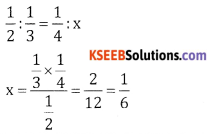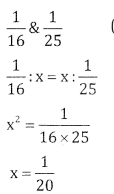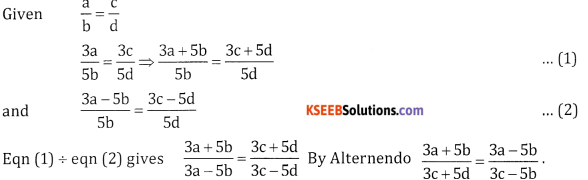# 2nd PUC Basic Maths Question Bank Chapter 7 Ratios and Proportions Ex 7.2

Students can Download Basic Maths Exercise 7.2 Questions and Answers, Notes Pdf, 2nd PUC Basic Maths Question Bank with Answers helps you to revise the complete Karnataka State Board Syllabus and score more marks in your examinations.

## Karnataka 2nd PUC Basic Maths Question Bank Chapter 7 Ratios and Proportions Ex 7.2

Part – A

2nd PUC Basic Maths Ratios and Proportions Ex 7.2 One Mark Questions and Answers ( 1 × 4 = 4)

Question 1.
State which of the following are proportions.
(1) 2 : 3 :: 6 : 9
(ii) 1 : 3 :: 4 : 15
(iii) 3 : 5 :: 6 : 15
(iv) 7 : 9 :: 14 : 18
(i) 2 × 9 = 3 × 6
18 = 18 It is a proportion

(ii) 1 × 15 ≠ 3 × 4
It is not a proportion

(iii) 3 × 15 ≠ 5 × 6
∴not a proportion

(iv) 7 × 18 = 9 × 14 ii
126 = 126 It is a proportionQuestion 2.
Find the fourth proportional to each of the following.
(1) 4, 5, 24
(ii) 6, 12, 15
(ii) 1.5, 4.5, 3.5
(iv) $$\frac{1}{2}, \frac{1}{3}, \frac{1}{4}$$
Let x be the fourth proportional
(i) 4 : 5 :: 24 : x
4x = 5 x 24 ⇒ x = $$\frac{5 \times 24}{4}$$ ⇒ X=30

(ii) 6 : 12 = 15 : x
⇒ x =$$\frac{12 \times 15}{6}$$ = 30 ⇒ x = 30

(iii) 1.5 : 4.5 = 3.5 : x
x = $$\frac{4.5 \times 3.5}{1.5}$$ = 10.5

(iv)Question 3.
Find the mean proportional to each of the following.
(i) 1 and 9
(ii) 2 and 8
(iii) $$\frac{1}{16}$$ and $$\frac{1}{25}$$
(iv) 0.8 and 1.8
Let x be the mean proportional

(i) 1&9
1 : x= x : 9
x2 = 9
x = 3

(ii) 2 & 8
2 : x = x : 8
x2 = 16
X = 4

(iii)(iv) 0.8 and 1.8
x2 = 0.8 x 1.8
x = 1.2Question 4.
Find the third proportional to each of the following,
(i) 4, 6
(ii) 3,12
(iii) 2.4, 3.6
(iv) 2$$\frac{2}{3}$$ , 4
Let x be the third proportional
(i) 4,6
4:6 = 6 : x
x = $$\frac{36}{4}$$ = 9

(ii) 3, 12
3 : 12 = 12 : x
x = $$\frac{12 \times 12}{3}$$ = 48

(iii) 2.4, 3.6
2.4:3.6 = 3.6 : x
x = $$\frac{3.6 \times 3.6}{2.4}$$ = 5.4

(iv) 2$$\frac{2}{3}$$ , 4
$$\frac{8}{3}$$:4 = 4 : x
2.4 : 3.6 = 3.6 : x
x = $$\frac{4 \times 4}{\frac{8}{3}}$$ = 6

Part – B and C

2nd PUC Basic Maths Ratios and Proportions Ex 7.2 Three Marks Questions and Answers ( 3 × 6 = 18)

Question 1.
If $$\frac{a}{b}=\frac{c}{d}$$ then prove that $$\frac{3 a+5 b}{3 c+5 d}=\frac{3 a-5 b}{3 c-5 d}$$Question 2.
If $$\frac{\sqrt{1+x}+\sqrt{1-x}}{\sqrt{1+x}-\sqrt{1-x}}=\frac{a}{b}$$find xQuestion 3.
Find the numbers which added to the numerator and denominator of 25 : 37 make it 5 : 6.
Let x be added to numerator and denominator of 25 : 37.
∴ $$\frac{25+x}{37+x}=\frac{5}{6}$$
150 + 6x = 185 + 5x
x = 185 – 150 = 35
∴ x = 35.

Question 4.
The ages of a father and his for son in the ratio 6:1. After 14 years their age will be in the ratio 8:3 what are their present ages?
Let the father’s age be 6x and Son’s age be x
After 14 years father’s age is 6x + 14 and Son’s is x + 14
Given $$\frac{6 x+14}{x+14}=\frac{8}{3}$$
18x + 42 = 8x + 112
10x = 70 ⇒ x = 7
∴ father’s age is 6x = 6 x 7 = 42 years and son’s age = 7 years.

Question 5.
Four numbers formed by adding 1, 5, 10 and 15 to a certain number are in proportion. Find the number?
Let the number be x
∴ 1 + x, 5 + x, 10 + x, 15 + x are in proportion
∴ (5 + x) (10 + x) = (1 + x) (15 + x)
50 + 10x + 5x + x2 = 15 + 15x + x + x2
⇒ x= 35Question 6.
A concrete mixture was made of cement, chips and sand. The ratio of the cement and chip is the same as that of chips and the sand. If 144 bags of cement and 225 bags of sand were used is the mixture. Find the
number of bags of chips used in the mixture.